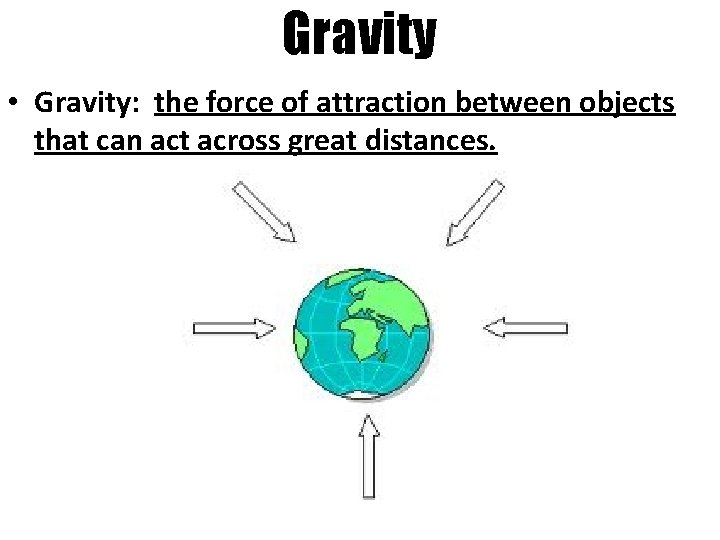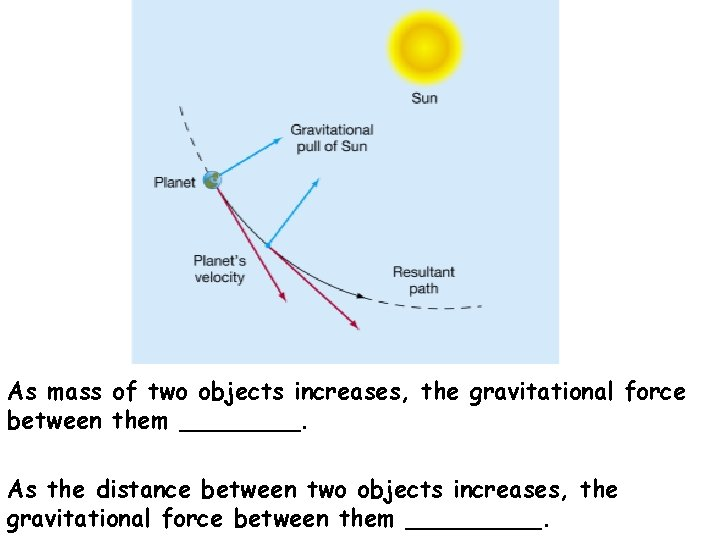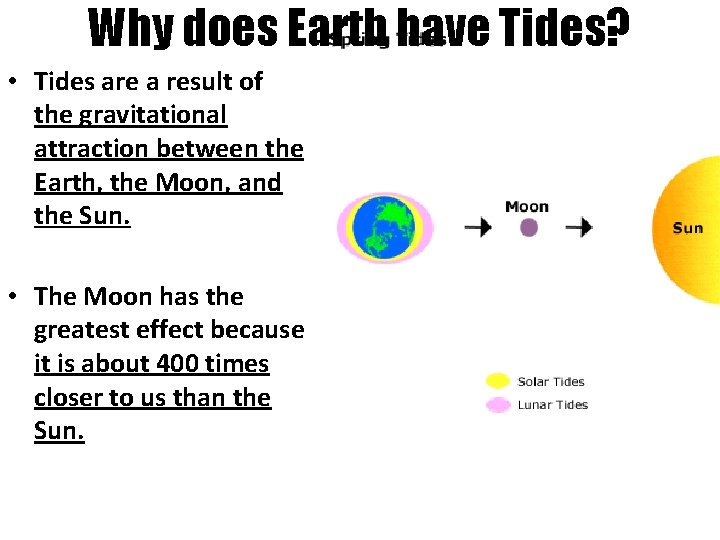# AIM How does gravity affect the movement of

• Slides: 13AIM: How does gravity affect the movement of planets?Gravity • Gravity: the force of attraction between objects that can act across great distances.• The Sun’s gravity keeps the planets in their orbits around it. • The Earth’s gravity keeps the Moon in its orbit.• The gravitational attraction between two objects depends on two things: 1. Distance between objects 2. Mass of the objectsWhy don’t planets just fall into the Sun? • British mathematician, Isaac Newton, explained how gravitational forces and inertia interact to produce orbital motion. 1. Universal Law of Gravitation: • As the mass of objects increases, gravitational attraction INCREASES. • As the distance between two objects increases, gravitational attraction DECREASES.2. Newton’s first law of motion: An object at rest will stay at rest, and an object in motion will stay in motion, unless something acts on it to change that motion. – Inertia: the tendency of an object to remain in motion along the same, straight path. AnimationAs mass of two objects increases, the gravitational force between them ____. As the distance between two objects increases, the gravitational force between them _____.How does gravity cause tides? • Tides: the cyclic rise and fall of ocean waters.Local Tides for Kings Point, NYWhy does Earth have Tides? • Tides are a result of the gravitational attraction between the Earth, the Moon, and the Sun. • The Moon has the greatest effect because it is about 400 times closer to us than the Sun.• As Earth rotates, the low and high tides follow the alignment of Earth and the moon. • The time between two high (or two low) tides is ideally about 12 hours and 25 minutes.